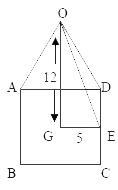# Sample Aptitude Questions of SML-ISUZU

1. A right pyramid stands on a square base of side10 cm. If the height of the pyramid is 12 cm, the area (in cm2) of its slant surface is
1. 520
2. 420
3. 360
4. 260As the side of Square base = 10
Then GE = 1/2 * 10 = 5
As Triangle OGE is a right angle triangle , so
OE2 = OG2 + GE2 = 144+25 = 169
hence, OE = 13
In triangle OCD, height of the triangle = OE = 13
DC = 10
Hence, the Area of Slant surface = 4* Area of triangle OCD ( as the pyramid is regular )
= 4 * (1/ 2 * 10 * 13) = 260cm 2
Hence, the answer is option D.
2. If each interior angle of a regular polygon is 150°, the number of sides of the polygon is
1. 8
2. 10
3. 15
4. None of these

Each interior angle in a regular polygon = (n-2) x 180/n
where, n is the no of sides.
Here, (n-2) x 180/n = 150
=> 180n - 360 = 150n
=> 30n = 360
=> n = 12
So, Ans. is option D.
1. If the Altitude of a right prism is 10 cm and its base is an equilateral triangle of side 12 cm, then its total surface area ( in cm2 ) is
1. 5 + 3√3
2. 36√3
3. 360
4. 72( 5 + √3 )Total Surface area = Perimeter of base x height
+ 2 x area of base
= 36 x 10 - 2 x √3/4 x 12 2
= 360 +72 √ 3
= 72(5+ √ 3)
so, as option D is correct.
2. The value of tan 10° tan 15° tan 75° tan 80° is
1. 0
2. 1
3. -1
4. 2

tan10 tan15 tan75 tan80
we know that  tan10 = tan(90 - 80) = cot 80o
and tan15 = tan( 90 - 75) = cot 75o
Therefore,tan10 tan15 tan75 tan80
= cot80 cot75 tan75 tan80
=1/tan80 x 1/tan75 x tan80 =1 =so,Answer is option B

3. The minimum value of 4 tan2θ + 9 cot2θ is equal to
1. 0
2. 5
3. 12
4. 13

Arithmetic mean(A.M) = Geometric mean(G.M)
Applying , we get
4 tan2Θ + 9 cot 2Θ /2 ≥ √ 4 tan2Θ 9 cot 2Θ
4 tan2Θ + 9 cot2Θ ≥ 2√ 36 tan 2Θ  cot 2Θ
≥ 2 x 6√tan 2Θ .  1 / tan 2Θ
≥  12 x 1
4 tan2Θ + 9 cot 2Θ ≥  12
So,minimum value is 12
4. If sin 7x = cos11x, then the value of tan 9x + cot 9x is
1. 1
2. 2
3. 3
4. 4

sin 7x =  cos 4x
=>sin (9x-2x) = cos(9x-2x)
=>sin 9x.cos 2x - cos9x.sin2x =cos9x.cos2x -sin 9x.sin 2x
=>sin 9x.cos 2x + sin 9x.sin 2x =cos9x.cos2x +cos9x.sin2x
=>sin 9x.(cos 2x + sin 2x) = cos 9x.(cos 2x +sin 2x)
=>sin 9x/cos 9x = 1
=>tan 9x = 1
Now,tan 9x+ cot 9x
= tan 9x + 1 /tan 9x
=  1 + 1/1 = 2
5. A person sets to cover a distance of 12 km in 45 minutes. If he covers of the distance in of time, then what is the speed in the remaining time?
1. 16 km/hr
2. 8 km/hr
3. 12 km/hr
4. 55 km/hr
5. 10 km/hr

Distance already covered = 3/2*12 = 9 km, Time spent = 2/3*45 min = 30 min
Distance left = (12 – 9) km = 3 km, Time left = (45 – 30) min = 15 min
Therfore, Required speed = 3/ 15/60 km/hr = 12 km/hr.
6. If a train 110 metres in length passes a man walking at the rate of 6 km/hr against it in 6 seconds, it will pass another man walking at the same speed in the same direction in time of
1. 91/3sec
2. 101/3sec
3. 8 sec
4. 6 sec
5. 71/3sec

Let the speed of the train = x km/hr. Relative speed = (x + 6) km/hr = (x + 6) X 15/8 m/sec
(x + 6)x 5/18x6 = 110, x = 60. Speed of train = 60 km/hr for 2nd person,
Relative speed = (60 – 6) km/hr = 54x5/18 m/sec = 15 m/hr. Time taken to cross 2nd person =110/15=22/3= 7 1/3 sec.
7. (91.66 % of 24 +3)1/2 = ? +2
1. 9
2. 5
3. 7
4. 8
5. 3